# scipy.spatial.distance.pdist¶

scipy.spatial.distance.pdist(X, metric='euclidean', p=2, w=None, V=None, VI=None)[source]

Pairwise distances between observations in n-dimensional space.

The following are common calling conventions.

1. Y = pdist(X, 'euclidean')

Computes the distance between m points using Euclidean distance (2-norm) as the distance metric between the points. The points are arranged as m n-dimensional row vectors in the matrix X.

2. Y = pdist(X, 'minkowski', p)

Computes the distances using the Minkowski distance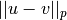(p-norm) where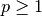.

3. Y = pdist(X, 'cityblock')

Computes the city block or Manhattan distance between the points.

4. Y = pdist(X, 'seuclidean', V=None)

Computes the standardized Euclidean distance. The standardized Euclidean distance between two n-vectors u and v is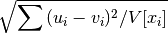V is the variance vector; V[i] is the variance computed over all the i’th components of the points. If not passed, it is automatically computed.

5. Y = pdist(X, 'sqeuclidean')

Computes the squared Euclidean distance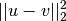between the vectors.

6. Y = pdist(X, 'cosine')

Computes the cosine distance between vectors u and v,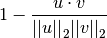where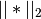is the 2-norm of its argument *, and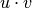is the dot product of u and v.

7. Y = pdist(X, 'correlation')

Computes the correlation distance between vectors u and v. This is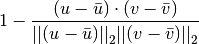where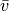is the mean of the elements of vector v, and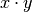is the dot product of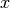and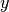.

8. Y = pdist(X, 'hamming')

Computes the normalized Hamming distance, or the proportion of those vector elements between two n-vectors u and v which disagree. To save memory, the matrix X can be of type boolean.

9. Y = pdist(X, 'jaccard')

Computes the Jaccard distance between the points. Given two vectors, u and v, the Jaccard distance is the proportion of those elements u[i] and v[i] that disagree where at least one of them is non-zero.

10. Y = pdist(X, 'chebyshev')

Computes the Chebyshev distance between the points. The Chebyshev distance between two n-vectors u and v is the maximum norm-1 distance between their respective elements. More precisely, the distance is given by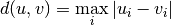1. Y = pdist(X, 'canberra')

Computes the Canberra distance between the points. The Canberra distance between two points u and v is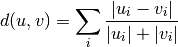1. Y = pdist(X, 'braycurtis')

Computes the Bray-Curtis distance between the points. The Bray-Curtis distance between two points u and v is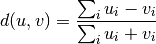1. Y = pdist(X, 'mahalanobis', VI=None)
Computes the Mahalanobis distance between the points. The Mahalanobis distance between two points u and v is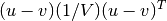where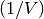(the VI variable) is the inverse covariance. If VI is not None, VI will be used as the inverse covariance matrix.
1. Y = pdist(X, 'yule')
Computes the Yule distance between each pair of boolean vectors. (see yule function documentation)
1. Y = pdist(X, 'matching')
Computes the matching distance between each pair of boolean vectors. (see matching function documentation)
1. Y = pdist(X, 'dice')
Computes the Dice distance between each pair of boolean vectors. (see dice function documentation)
1. Y = pdist(X, 'kulsinski')
Computes the Kulsinski distance between each pair of boolean vectors. (see kulsinski function documentation)
1. Y = pdist(X, 'rogerstanimoto')
Computes the Rogers-Tanimoto distance between each pair of boolean vectors. (see rogerstanimoto function documentation)
1. Y = pdist(X, 'russellrao')
Computes the Russell-Rao distance between each pair of boolean vectors. (see russellrao function documentation)
1. Y = pdist(X, 'sokalmichener')
Computes the Sokal-Michener distance between each pair of boolean vectors. (see sokalmichener function documentation)
1. Y = pdist(X, 'sokalsneath')
Computes the Sokal-Sneath distance between each pair of boolean vectors. (see sokalsneath function documentation)
1. Y = pdist(X, 'wminkowski')
Computes the weighted Minkowski distance between each pair of vectors. (see wminkowski function documentation)
1. Y = pdist(X, f)

Computes the distance between all pairs of vectors in X using the user supplied 2-arity function f. For example, Euclidean distance between the vectors could be computed as follows:

dm = pdist(X, lambda u, v: np.sqrt(((u-v)**2).sum()))


Note that you should avoid passing a reference to one of the distance functions defined in this library. For example,:

dm = pdist(X, sokalsneath)


would calculate the pair-wise distances between the vectors in X using the Python function sokalsneath. This would result in sokalsneath being called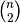times, which is inefficient. Instead, the optimized C version is more efficient, and we call it using the following syntax.:

dm = pdist(X, 'sokalsneath')

Parameters : X : ndarray An m by n array of m original observations in an n-dimensional space. metric : string or function The distance metric to use. The distance function can be ‘braycurtis’, ‘canberra’, ‘chebyshev’, ‘cityblock’, ‘correlation’, ‘cosine’, ‘dice’, ‘euclidean’, ‘hamming’, ‘jaccard’, ‘kulsinski’, ‘mahalanobis’, ‘matching’, ‘minkowski’, ‘rogerstanimoto’, ‘russellrao’, ‘seuclidean’, ‘sokalmichener’, ‘sokalsneath’, ‘sqeuclidean’, ‘yule’. w : ndarray The weight vector (for weighted Minkowski). p : double The p-norm to apply (for Minkowski, weighted and unweighted) V : ndarray The variance vector (for standardized Euclidean). VI : ndarray The inverse of the covariance matrix (for Mahalanobis). Y : ndarray Returns a condensed distance matrix Y. For eachand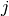(where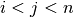), the metric dist(u=X[i], v=X[j]) is computed and stored in entry ij.

squareform
converts between condensed distance matrices and square distance matrices.

Notes

See squareform for information on how to calculate the index of this entry or to convert the condensed distance matrix to a redundant square matrix.

#### Previous topic

scipy.spatial.distance.yule

#### Next topic

scipy.spatial.distance.cdist